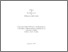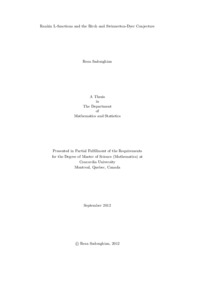Title:

Rankin L-functions and the Birch and Swinnerton-Dyer Conjecture

Sadoughianzadeh, Reza (2012) Rankin L-functions and the Birch and Swinnerton-Dyer Conjecture. Masters thesis, Concordia University.Preview
Text (application/pdf)
691kB

Abstract

In this thesis we use Rankin's method to evaluate the central critical value of the L-series attached to an elliptic curve E over Q and certain odd irreducible 2-dimensional Artin representation tau: G_Q --> GL_2(C). The motivation for this study is the twisted BSD conjecture which states that:

For any continuous irreducible finite-dimensional complex representation tau of Gal(Qbar}/Q), we have:
ord_{s=1}L(\rho_E tensor \tau,s)= multiplicity of tau in C tensor E(K)
where E is an elliptic curve over Q, K is the fixed field of Ker(tau) and rho_E is the 2-dimensional l-adic Galois representation attached to the elliptic curve E, namely, the p-adic Tate module of E.

Deligne and Serre proved that there is a correspondence between the modular forms of weight 1 and certain two-dimensional Galois representations. In the twisted BSD conjecture, we put tau=rho_g where \rho_g:Gal(K/Q)-->GL_2(C) is an odd, continuous and irreducible Galois representation arising from a cusp form g belonging to S_1(Gamma_0(N),\chi). Using the Rankin method, we compute the constant term of L(\rho_{g}\otimes \rho_{E},s) at s=1. Thus, if we assume the BSD, we deduce:

L(rho_E tensor rho_g,1)= 0 ---> Hom_{G_{Q}} (rho_g, C tensor E(K))<> 0.

In this case, one can say that r_{K}(E) >= 2 .

We present some tables of computations we did with Sage for different modular forms g and elliptic curves E.

Divisions: Concordia University > Faculty of Arts and Science > Mathematics and Statistics Thesis (Masters) Sadoughianzadeh, Reza Concordia University M. Sc. Mathematics 14 September 2012 Iovita, Adrian and Darmon, Henri 974751 REZA SADOUGHIANZADEH 20 Dec 2012 20:54 18 Jan 2018 17:38
All items in Spectrum are protected by copyright, with all rights reserved. The use of items is governed by Spectrum's terms of access.

Repository Staff Only: item control page

Back to top